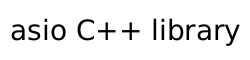### Gettable socket option requirements

A type `X` meets the `GettableSocketOption` requirements if it satisfies the requirements listed below.

In the table below, `a` denotes a (possibly const) value of type `X`, `b` denotes a value of type `X`, `p` denotes a (possibly const) value that meets the `Protocol` requirements, and `s` denotes a (possibly const) value of a type that is convertible to `size_t` and denotes a size in bytes.

Table 20. GettableSocketOption requirements for extensible implementations

expression

type

assertion/note
pre/post-conditions

`a.level(p)`

`int`

Returns a value suitable for passing as the level argument to POSIX `getsockopt()` (or equivalent).

`a.name(p)`

`int`

Returns a value suitable for passing as the option_name argument to POSIX `getsockopt()` (or equivalent).

`b.data(p)`

`void*`

Returns a pointer suitable for passing as the option_value argument to POSIX `getsockopt()` (or equivalent).

`a.size(p)`

`size_t`

Returns a value suitable for passing as the option_len argument to POSIX `getsockopt()` (or equivalent), after appropriate integer conversion has been performed.

`b.resize(p,s)`

post: ```b.size(p) == s```.
Passed the value contained in the option_len argument to POSIX `getsockopt()` (or equivalent) after successful completion of the function. Permitted to throw an exception if the socket option object `b` does not support the specified size.